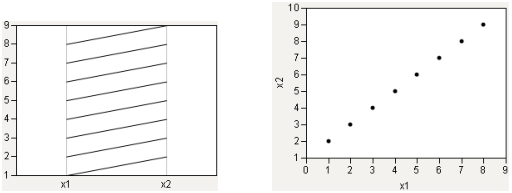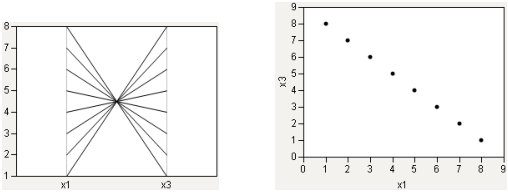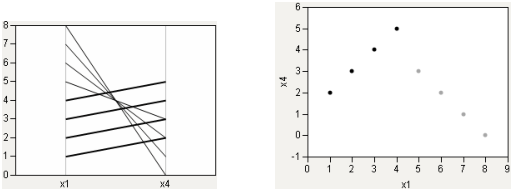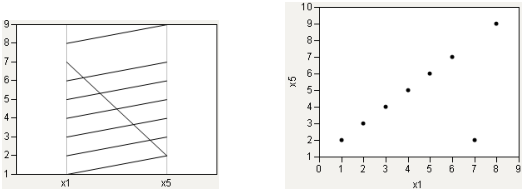Essential Graphing > Parallel Plots > The Parallel Plot > Interpreting Parallel Plots
Publication date: 08/13/2020

## Interpreting Parallel Plots

To help you interpret parallel plots, compare the parallel plot with a scatterplot. In each of the following figures, the parallel plot appears on the left, and the scatterplot appears on the right.

#### Strong Positive Correlation

The following relationship shows a strong positive correlation. Notice the coherence of the lines in the parallel plot.

Figure 7.6 Strong Positive Correlation#### Strong Negative Correlation

A strong negative correlation, by contrast, shows a narrow neck in the parallel plot.

Figure 7.7 Strong Negative Correlation#### Collinear Groups

Now, consider a case that encompasses both situations: two groups, both strongly collinear. One has a positive slope, the other has a negative slope. In Figure 7.8, the positively sloped group is highlighted.

Figure 7.8 Collinear Groups: Parallel Plot and Scatterplot#### Single Outlier

Finally, consider the case of a single outlier. The parallel plot shows a general coherence among the lines, with a noticeable exception.

Figure 7.9 Single Outlier: Parallel Plot and ScatterplotRelated Information